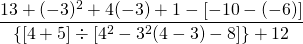Chapter 1: Algebra Review

# 1.3 Order of Operations (Review)

When simplifying expressions, it is important to do so in the correct order. Consider the problem 2 + 5 ⋅ 3 done two different ways:

Method 1: Add first Method 2: Multiply first
Add: 2 + 5 ⋅ 3 Multiply: 2 + 5 ⋅ 3
Multiply: 7 ⋅ 3 Add: 2 + 15
Solution: 21 Solution: 17

The previous example illustrates that if the same problem is done two different ways, it will result in two different solutions. However, only one method can be correct. It turns out the second method is the correct one. The order of operations ends with the most basic of operations, addition (or subtraction). Before addition is completed, do all repeated addition, also known as multiplication (or division). Before multiplication is completed, do all repeated multiplication, also known as exponents. When something is supposed to be done out of order, to make it come first, put it in parentheses (or grouping symbols). This list, then, is the order of operations used to simplify expressions.

Key Takeaways: Order of Operations

1st Brackets (Grouping)

2nd Exponents

3rd Multiplication and Division (Left to Right)

4th Addition and Subtraction (Left to Right)

Multiplication and division are on the same level because they are the same operation (division is just multiplying by the reciprocal). This means multiplication and division must be performed from left to right. Therefore, division will come first in some problems, and multiplication will come first in others. The same is true for adding and subtracting (subtracting is just adding the opposite).

Often, students use the word BEMDAS to remember the order of operations, as the first letter of each operation creates the word (written as B E MD AS). Remember BEMDAS to ensure that multiplication and division are done from left to right (same with addition and subtraction).

Example 1.3.1

Evaluate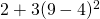using the order of operations.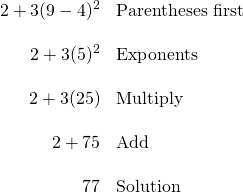It is very important to remember to multiply and divide from left to right!

Example 1.3.2

Evaluate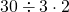using the order of operations.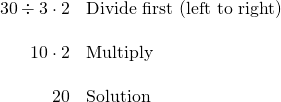If there are several sets of parentheses in a problem, start with the innermost set and work outward. Inside each set of parentheses, simplify using the order of operations. To make it easier to know which left parenthesis goes with which right parenthesis, different types of grouping symbols will be used, such as braces { }, brackets [ ], and parentheses ( ). These all do the same thing: they are grouping symbols and must be evaluated first.

Example 1.3.3

Evaluate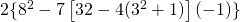using the order of operations.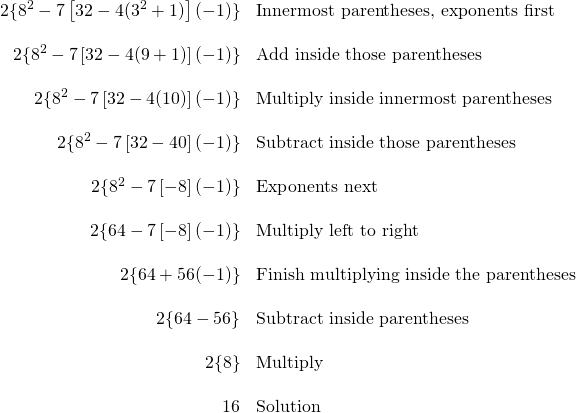As Example 1.3.3 illustrates, it can take several steps to complete a problem. The key to successfully solving order of operations problems is to take the time to show your work and do one step at a time. This will reduce the chance of making a mistake along the way.

There are several types of grouping symbols that can be used besides parentheses, brackets, and braces. One such symbol is a fraction bar. The entire numerator and the entire denominator of a fraction must be evaluated before reducing. Once the fraction is reduced, the numerator and denominator can be simplified at the same time.

Example 1.3.4

Evaluate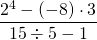using the order of operations.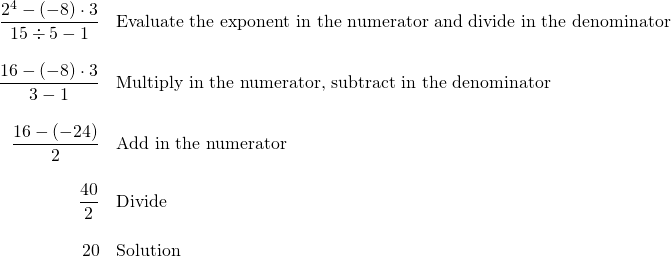Another type of grouping symbol is the absolute value. Everything inside a set of absolute value brackets must be evaluated, just as if it were a normal set of parentheses. Then, once the inside is completed, take the absolute value—or distance from zero—to make the number positive.

Example 1.3.5

Evaluate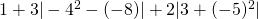using the order of operations.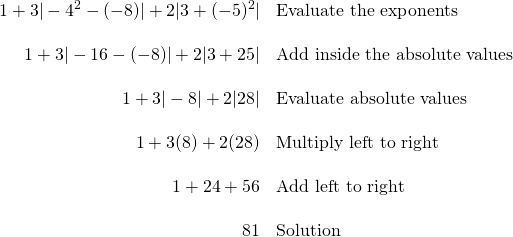Key Takeaways: Exponents

The above example also illustrates an important point about exponents:

• Exponents are only considered to be on the number they are attached to.
• This means that, in the expression −42, only the 4 is squared, giving us −(42) or −16.
• But when the negative is in parentheses, such as in (−5)2, the negative is part of the number and is also squared, giving a positive solution of 25.

# Questions

For questions 1 to 24, reduce and solve the following expressions.

1.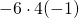2.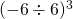3.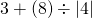4.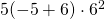5.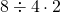6.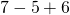7.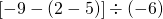8.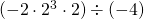9.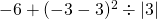10.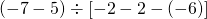11.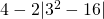12.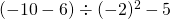13.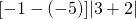14.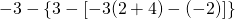15.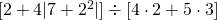16.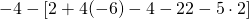17.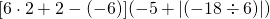18.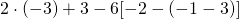19.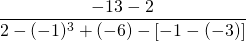20.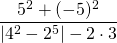21.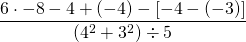22.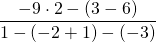23.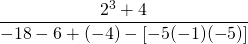24.